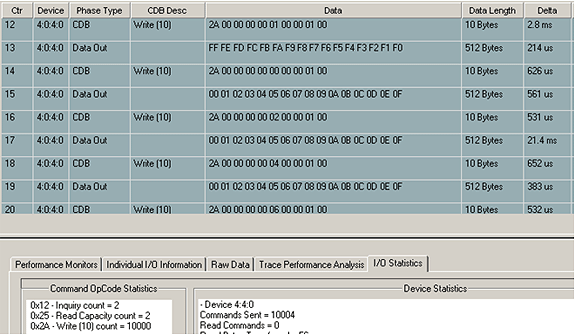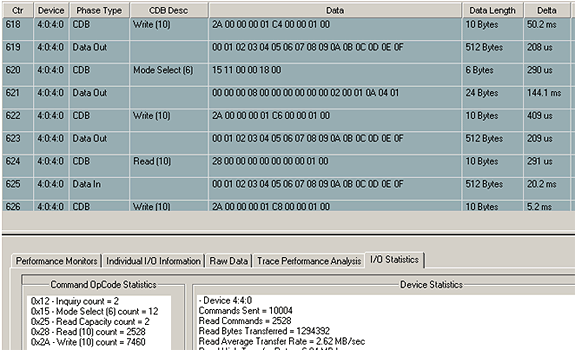# Introduction to the Command Probability Sequencer

### The Command Probability Sequencer (CPS) is a new API in VCPSSL v8.2.0 that allows you to define a set of commands that will be issued; each command has a specified probability it will be chosen for execution.  This new API is quite complex and has many features – we will introduce the features by way of examples.

As a first example, suppose you wanted to issue a lot of writes and reads, but every now and then issue a different command (like Log Sense command for example).  And suppose you have a further requirement that this Log Sense command be issue about every 1000th I/O.  The Command Probability Sequencer is perfect for implementing the above test scenario.  Although the coding example below at first looks daunting, but for now focus on the following items: we are setting up three user-defined commands “Write”, “Read”, and “Log Sense” and we need to specify such information as whether data is going to/from the drive (the “nDataDir” field), and how much data needs to be transfer (the “nTransferLen” field).  Here’s the code for our first example – focus on the lines of code in RED which have the “nDataDir” and “nTransferLength” parameters, and the actual definition of the commands.

const int c_nLenOfArray = 3;

DMM_UserDefinedCDB arrOfCDB[c_nLenOfArray];

double arrOfProb[c_nLenOfArray];

int nIndex;

const long c_lNumberOfIOToIssue = 10000;

//Set up the “Write” command (Write is opcode 0x2A)

BYTE cCDB0[] = {0x2A,0,0,0,0,0,0,0,1,0};

nIndex = 0;

memcpy(arrOfCDB[nIndex].cCDBBytes,cCDB0,10);

arrOfCDB[nIndex].nCDBLength = 10;

arrOfCDB[nIndex].nTransferLength = 512;

BYTE cCDB1[] = {0x28,0,0,0,0,0,0,0,1,0};

nIndex = 1;

memcpy(arrOfCDB[nIndex].cCDBBytes,cCDB1,10);

arrOfCDB[nIndex].nCDBLength = 10;

arrOfCDB[nIndex].nTransferLength = 512;

//Set up the “Log Sense” command (Log Sense opcode is 0x4D)

BYTE cCDB2[] = {0x4D,0,0×40,0,0,0,0,0,0,0×80,0};

nIndex = 2;

memcpy(arrOfCDB[nIndex].cCDBBytes,cCDB2,10);

arrOfCDB[nIndex].nCDBLength = 10;

arrOfCDB[nIndex].nTransferLength = 128;

//Now define the probabilities for each of the three commands

arrOfProb = 0.4995;  //this is the probability for the “Write” command

arrOfProb = 0.4995;  //this is the probability for the “Read” command

arrOfProb = 0.001;  //.001 probability means the Log Sense command will be issued

//with probability .001 (i.e. every 1000th I/O)

arrOfProb,

c_nLenOfArray,

c_lNumberOfIOToIssue);

In the example above our set of commands to issue is 3 (hence we set c_nLenOfArry to 3) and the number of commands to issue is 10000 (hence we set c_lNumberOfIOToIssue to 10000).  Notice also in the call to API VCSCSIAddDiskComProbSeqTest we pass in two arrays: the first is the sequence of commands, and the second is the sequence containing the probabilities for these commands.

NOTE: The sum of your probabilities must be exactly 1.0 (or 100%).  Notice in our example the sum of the probabilities .4995, .4995, and .001 is exactly 1.0

Here is a BAM (Bus Analyzer Module) output from the above test:As you can see in the trace, there are Write, Log Sense, and Read commands.  In the lower portion of the trace, on the I/O Statistics page, it shows the number and type of commands that went out.  There were 5019 Read commands, 4966 Write commands, and 15 Log Sense commands (note that 5019 + 4966 + 15 = 10000, which is the number of commands we specified to CPS to issue).  From this particular run, we see that the Read commands formed 5019/10000 = 50.19% of the commands, the Write commands formed 4966/10000 = 49.66% of the commands, and the Log Sense commands formed 15/10000 = 0.15%.  These percentages are almost exactly the probabilities we specified to CPS.

## SECOND EXAMPLE: Writing Every Even LBA With An Incrementing Pattern and Every Odd LBA with a Decrementing Pattern

In this example we will cover two more features of the CPS – namely how to set the data pattern, and how to adjust your write and read (and other commands) automatically.  To see why you would want to adjust the write command, suppose for the moment you did not adjust the command.  Then every single time we issued the command we would be writing to the exact same location on the drive (in example 1, we would be writing to LBA 0 every single time).  To allow adjusting the location the command writes to, we have introduced the “nGap” parameter.  The nGap parameter tells CPS how much to adjust the location of the write command.  For example, if nGap is 7 then successive writes would go to LBAs 0, 7, 14, 21, and so forth.

In order to write every even LBA (i.e. the LBAs 0, 2, 4, 6, 8, 10, ….) we will need nGap to be exactly 2.

So here’s how to see up the above type of test – focus on the lines of code in RED which have the “eTestPattern” and “nGap” parameters.

const int c_nLenOfArray = 2;

DMM_UserDefinedCDB arrOfCDB[c_nLenOfArray];

double arrOfProb[c_nLenOfArray];

int nIndex;

const long c_lNumberOfIOToIssue = 10000;

//Set up the “Write” command that writes to even LBA, with Incrementing pattern

BYTE cCDB0[] = {0x2A,0,0,0,0,0,0,0,1,0};

nIndex = 0;

memcpy(arrOfCDB[nIndex].cCDBBytes,cCDB0,10);

arrOfCDB[nIndex].nCDBLength = 10;

arrOfCDB[nIndex].nTransferLength = 512;

arrOfCDB[nIndex].eTestPattern = eIncrementing;

arrOfCDB[nIndex].nGap = 2;

//Set up the “Write” command that writes to odd LBA, with Decrementing pattern

BYTE cCDB1[] = {0x2A,0,0,0,0,1,0,0,1,0};

nIndex = 1;

memcpy(arrOfCDB[nIndex].cCDBBytes,cCDB1,10);

arrOfCDB[nIndex].nCDBLength = 10;

arrOfCDB[nIndex].nTransferLength = 512;

arrOfCDB[nIndex].eTestPattern = eDecrementing;

arrOfCDB[nIndex].nGap = 2;

//Now define the probabilities for these two commands.  There’s no reason you have

//to make the probabilities the same.

arrOfProb = 0.71;  //71% of the time we’ll be writing to the even LBA

arrOfProb = 0.29;  //29% of the time we’ll be writing to the odd LBA

arrOfProb,

c_nLenOfArray,

c_lNumberOfIOToIssue);

Here is a BAM (Bus Analyzer Module) output from the above test:Notice in the BAM trace, the first Write command writes a decrementing pattern (to LBA 1), while the next three Write commands write an incrementing pattern (to LBA 0, 2, 4).  Notice the “spacing” between the Write commands with incrementing pattern – they are exactly two blocks apart, which is exactly the nGap value we specified to CPS.

## THIRD EXAMPLE: SENDING SPECIALIZE DATA TO THE DRIVE

In this third example we show you how to send unique data to a drive in a “Mode Select” command.  We will be setting the AWRE (“Automatic Write Reallocation Enabled” bit to 0 on the “Error Recovery” mode page).  This Mode Select will be done only with probability one-tenth of one percent (i.e. probability .001).

const int c_nLenOfArray = 3;

DMM_UserDefinedCDB arrOfCDB[c_nLenOfArray];

double arrOfProb[c_nLenOfArray];

int nIndex;

const long c_lNumberOfIOToIssue = 10000;

//Do a Mode-Select (Mode-Select has opcode 0x15)

BYTE cCDB0[] = {0x15,0x11,0,0,0×18,0};

BYTE cModeSelectBuffer = {0,0,0,0×08,0,0,0,0,0,0,2,0,1,0x0a,4,1,0,0,0,0,1,0,0,0};

nIndex = 0;

memcpy(arrOfCDB[nIndex].cCDBBytes,cCDB0,6);

arrOfCDB[nIndex].nCDBLength = 6;

arrOfCDB[nIndex].nTransferLength = 24;

//Set up the “Write” command (Write is opcode 0x2A)

BYTE cCDB1[] = {0x2A,0,0,0,0,0,0,0,1,0};

nIndex = 1;

memcpy(arrOfCDB[nIndex].cCDBBytes,cCDB1,10);

arrOfCDB[nIndex].nCDBLength = 10;

arrOfCDB[nIndex].nTransferLength = 512;

BYTE cCDB2[] = {0x28,0,0,0,0,0,0,0,1,0};

nIndex = 2;

memcpy(arrOfCDB[nIndex].cCDBBytes,cCDB2,10);

arrOfCDB[nIndex].nCDBLength = 10;

arrOfCDB[nIndex].nTransferLength = 512;

//Now define the probabilities for these two commands.  There’s no reason you have

//to make the probabilities the same.

arrOfProb = 0.001;  // .1% of the time we issue mode-select

arrOfProb = 0.4995;

arrOfProb = 0.4995;

arrOfProb,

c_nLenOfArray,

c_lNumberOfIOToIssue);

Here is a BAM (Bus Analyzer Module) output from the above test:## DEFINITIONS OF THE PARAMETERS FOR EACH COMMAND:

Here is the data structure that you must fill out for each command you want to set up for CPS:

struct _DMM_UserDefinedCDB

{

BOOL bValid;

eUSER_DEFINED_TYPES eUserDefinedType;

char cCDBBytes;

int nCDBLength;

int nTimeout;

int nTransferLength;

int nAmtDataToLogfile;

char cDataOutFile[MAX_PATH];

ePATTERN_TYPE eTestPattern;

BOOL bCompare;

int nGap;

int nSeed;

}

 bValid: Set it to TRUE eUserDefinedType: Set it to eScsiCDB cCDBBytes: copy the particular command to this field (must be 16 bytes or less) nCDBLength: set this to the length of your command nDataDir: set this to 0 if data is going TO the drive, and set it to 1 if data is coming BACK from the drive.  NOTE: If no data is being transferred, set it to 0. 0 is the default value nTimeout: set this to the desired timeout for the command (default value is 30) nTransferLength: set this to how much data is to be transferred.  If no data is being transferred then set this field to 0 pPayloadDataToDrive: set this pointer to the starting address of the buffer containing the data to be shipped to the drive.  If there is no data to be shipped to the drive then set this field to NULL (which is the default value). cDataOutFile: set byte 0 to ‘?’ – this is the default eTestPattern: set this field to the desired  pattern.  For a list of available patterns see VCPSSLImports.h and enum ePATTERN_TYPE. bCompare: Set this field to TRUE if the command is receiving data from the drive (for example a “Read” command).  Otherwise set it to FALSE (which is the default). The data coming back from the drive will be compared to whatever is in the eTestPattern field nGap: for Write and Read commands, set this field to the number of blocks you want between each successive commands

## RETURN CODES AND POSSIBLE PROBLEMS WITH USING VCSCSIAddDiskComProbSeq API:

This API returns TRUE to mean adding of the test to your sequence was done;

It returns FALSE if any problem occurred.

Reasons for getting a return code of FALSE from API VCSCSIAddDiskComProbSeq:

1. The number of commands in your sequence is too long.  Resolution: Make sure there are at most 1000 commands in your sequence
2. The sum of the probabilities for all your commands does not equal 1.0.  Resolution:  Make sure that the probabilities do in fact add up to 1.0 – it is very easy to “misplace” a decimal point or incorrectly input numbers.  Also, input the numbers as, for example, .25 (NOT as 25).